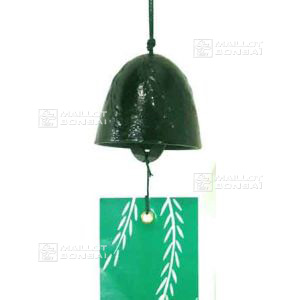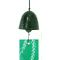##### The Japanese Bonsai specialist
Direct order Contact Help / Services Newsletter# Japanese cast iron swallows wind bell g48

› Wind bells and chimesref. : 4661

10,00

This item is temporarily unavailableNotify availability

###### Description

5 * 4.7 cm. Black wind bell embossed with swallow birds. Hang in the garden or next to the house for a soft, relaxing sound. The Tanzaku paper string features a graphic pine needle design. Made of durable Nambu cast iron.

#wind 5.4 #bell 3.4 #iron 3.4 #cast 3.4 #swallows 2.8 #japanese 2.8 #chimes 2.6 #bells 2.5 #embossed 1.8 #features 1.8

Formule
(( ROUND((CHAR_LENGTH(b.article_nom)-CHAR_LENGTH(REPLACE(b.article_nom, 'bell', '')))/LENGTH('bell')) + ROUND((CHAR_LENGTH(b.article_description)-CHAR_LENGTH(REPLACE(b.article_description, 'bell', '')))/LENGTH('bell')) ) * 3.4) + (( ROUND((CHAR_LENGTH(b.article_nom)-CHAR_LENGTH(REPLACE(b.article_nom, 'cast', '')))/LENGTH('cast')) + ROUND((CHAR_LENGTH(b.article_description)-CHAR_LENGTH(REPLACE(b.article_description, 'cast', '')))/LENGTH('cast')) ) * 3.4) + (( ROUND((CHAR_LENGTH(b.article_nom)-CHAR_LENGTH(REPLACE(b.article_nom, 'wind', '')))/LENGTH('wind')) + ROUND((CHAR_LENGTH(b.article_description)-CHAR_LENGTH(REPLACE(b.article_description, 'wind', '')))/LENGTH('wind')) ) * 3.4) + (( ROUND((CHAR_LENGTH(b.article_nom)-CHAR_LENGTH(REPLACE(b.article_nom, 'iron', '')))/LENGTH('iron')) + ROUND((CHAR_LENGTH(b.article_description)-CHAR_LENGTH(REPLACE(b.article_description, 'iron', '')))/LENGTH('iron')) ) * 3.4) + (( ROUND((CHAR_LENGTH(b.article_nom)-CHAR_LENGTH(REPLACE(b.article_nom, 'japanese', '')))/LENGTH('japanese')) + ROUND((CHAR_LENGTH(b.article_description)-CHAR_LENGTH(REPLACE(b.article_description, 'japanese', '')))/LENGTH('japanese')) ) * 2.8) + (( ROUND((CHAR_LENGTH(b.article_nom)-CHAR_LENGTH(REPLACE(b.article_nom, 'swallows', '')))/LENGTH('swallows')) + ROUND((CHAR_LENGTH(b.article_description)-CHAR_LENGTH(REPLACE(b.article_description, 'swallows', '')))/LENGTH('swallows')) ) * 2.8) + (( ROUND((CHAR_LENGTH(b.article_nom)-CHAR_LENGTH(REPLACE(b.article_nom, 'embossed', '')))/LENGTH('embossed')) + ROUND((CHAR_LENGTH(b.article_description)-CHAR_LENGTH(REPLACE(b.article_description, 'embossed', '')))/LENGTH('embossed')) ) * 1.8) + (( ROUND((CHAR_LENGTH(b.article_nom)-CHAR_LENGTH(REPLACE(b.article_nom, 'features', '')))/LENGTH('features')) + ROUND((CHAR_LENGTH(b.article_description)-CHAR_LENGTH(REPLACE(b.article_description, 'features', '')))/LENGTH('features')) ) * 1.8) + (( ROUND((CHAR_LENGTH(b.article_nom)-CHAR_LENGTH(REPLACE(b.article_nom, 'relaxing', '')))/LENGTH('relaxing')) + ROUND((CHAR_LENGTH(b.article_description)-CHAR_LENGTH(REPLACE(b.article_description, 'relaxing', '')))/LENGTH('relaxing')) ) * 1.8) + (( ROUND((CHAR_LENGTH(b.article_nom)-CHAR_LENGTH(REPLACE(b.article_nom, 'swallow', '')))/LENGTH('swallow')) + ROUND((CHAR_LENGTH(b.article_description)-CHAR_LENGTH(REPLACE(b.article_description, 'swallow', '')))/LENGTH('swallow')) ) * 1.7)

## Secure payment## Delivery

Our logistic partners :04 74 55 23 48
Pépinière MAILLOT-BONSAÏ
Le Bois Frazy
01990 RELEVANT - FRANCE
on appointment